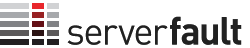## User count and total reputation for various reputation levels on Meta

2

(click on this box to dismiss)

Lists the total number of users, the total reputation, the average reputation, and site composition for categories of users on the Meta site.Q&A for system and network administrators

``````DECLARE @TotalUsers money --Money type makes it easy to group digits
DECLARE @TotalReputation money
DECLARE @Results table(Category varchar(18), Users money, Sum_Reputation money, Avg_Reputation money, Pct_Users decimal(6,1), Pct_Reputation decimal(6,1));

SELECT @TotalUsers = COUNT(ID), @TotalReputation = SUM(Reputation) from Users

Insert @Results(Category, Users, Sum_Reputation, Avg_Reputation, Pct_Users, Pct_Reputation)
Select 'All', @TotalUsers, @TotalReputation, @TotalReputation/@TotalUsers, 100, 100
Union ALL
Select 'Rep>1', COUNT(Id), SUM(Reputation), AVG(Convert(money,Reputation)), COUNT(ID) / @TotalUsers *100, SUM(Reputation) / @TotalReputation *100 FROM Users WHERE Reputation > 1
Union ALL
Select 'Rep>10', COUNT(Id), SUM(Reputation), AVG(Convert(money,Reputation)), COUNT(ID) / @TotalUsers *100, SUM(Reputation) / @TotalReputation *100 FROM Users WHERE Reputation > 10
Union ALL
Select 'Rep>100', COUNT(Id), SUM(Reputation), AVG(Convert(money,Reputation)), COUNT(ID) / @TotalUsers *100, SUM(Reputation) / @TotalReputation *100 FROM Users WHERE Reputation > 100
Union ALL
Select 'Rep>1,000', COUNT(Id), SUM(Reputation), AVG(Convert(money,Reputation)), COUNT(ID) / @TotalUsers *100, SUM(Reputation) / @TotalReputation *100 FROM Users WHERE Reputation > 1000
Union ALL
Select 'Rep>10,000', COUNT(Id), SUM(Reputation), AVG(Convert(money,Reputation)), COUNT(ID) / @TotalUsers *100, SUM(Reputation) / @TotalReputation *100 FROM Users WHERE Reputation > 10000
Union ALL
Select 'Rep>100,000', COUNT(Id), SUM(Reputation), AVG(Convert(money,Reputation)), COUNT(ID) / @TotalUsers *100, SUM(Reputation) / @TotalReputation *100 FROM Users WHERE Reputation > 100000
Union ALL
Select 'Rep<10', COUNT(Id), SUM(Reputation), AVG(Convert(money,Reputation)), COUNT(ID) / @TotalUsers *100, SUM(Reputation) / @TotalReputation *100 FROM Users WHERE Reputation < 10
Union ALL
Select 'Rep<100', COUNT(Id), SUM(Reputation), AVG(Convert(money,Reputation)), COUNT(ID) / @TotalUsers *100, SUM(Reputation) / @TotalReputation *100 FROM Users WHERE Reputation < 100
Union ALL
Select 'Rep<1,000', COUNT(Id), SUM(Reputation), AVG(Convert(money,Reputation)), COUNT(ID) / @TotalUsers *100, SUM(Reputation) / @TotalReputation *100 FROM Users WHERE Reputation < 1000
Union ALL
Select 'Rep<10,000', COUNT(Id), SUM(Reputation), AVG(Convert(money,Reputation)), COUNT(ID) / @TotalUsers *100, SUM(Reputation) / @TotalReputation *100 FROM Users WHERE Reputation < 10000
Union ALL
Select '[10..100]', COUNT(Id), SUM(Reputation), AVG(Convert(money,Reputation)), COUNT(ID) / @TotalUsers *100, SUM(Reputation) / @TotalReputation *100 FROM Users WHERE Reputation between 10 and 100
Union ALL
Select '[100..1,000]', COUNT(Id), SUM(Reputation), AVG(Convert(money,Reputation)), COUNT(ID) / @TotalUsers *100, SUM(Reputation) / @TotalReputation *100 FROM Users WHERE Reputation between 100 and 1000
Union ALL
Select '[1,000..10,000]', COUNT(Id), SUM(Reputation), AVG(Convert(money,Reputation)), COUNT(ID) / @TotalUsers *100, SUM(Reputation) / @TotalReputation *100 FROM Users WHERE Reputation between 1000 and 10000

Select Category, Parsename(Convert(VarChar(50),Users,1),2) as [Users], Parsename(Convert(VarChar(50),Sum_Reputation,1),2) as [Total Rep], Convert(VarChar(50), Avg_Reputation,1) as [Avg Rep], Convert(varchar(10), Pct_Users) + '%' as [% Users], Convert(varchar(10), Pct_Reputation) + '%' as [% Rep]  from @Results``````

### Enter Parameters

Options:
-Hold tight while we fetch your results
:records returned in :time ms:cached